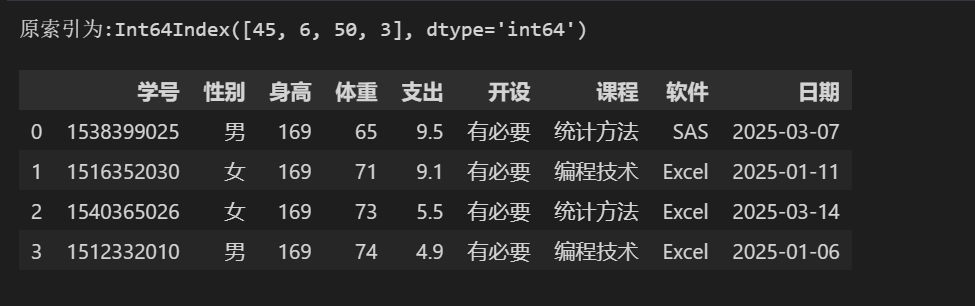Pandas 的主要数据结构是 Series （一维数据）与 DataFrame（二维数据），是一个提供高性能、易于使用的数据结构和数据分析工具。

⭐ 文件的基本操作

```# 导入模块
import pandas as pd
import numpy as np```

```# 读取文件```# 查看数据 （数据类型，是否有空值）
stu.info()```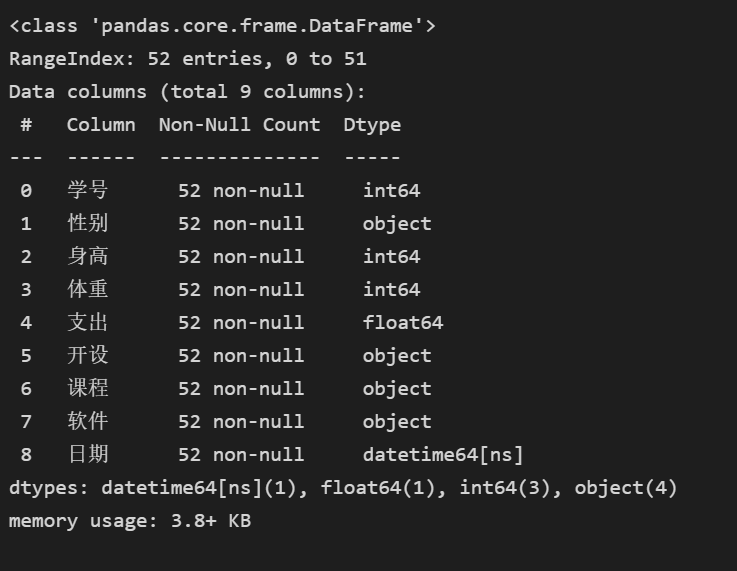```# 转换数据类型
stu['日期'] = stu['日期'].astype('str')
stu.info()```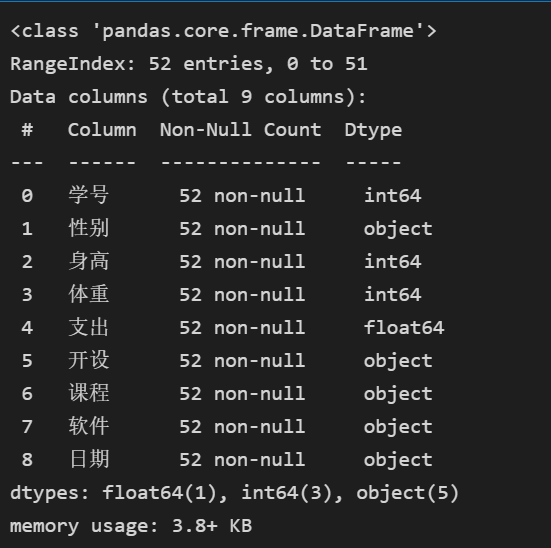⭐ 切片操作

```# iloc or loc切片 (学号，身高，体重）
stu.iloc[:,[0,2,3]]  # 获取学号,身高,体重，所有行信息
stu.loc[5:10,['学号','身高','体重'] ]```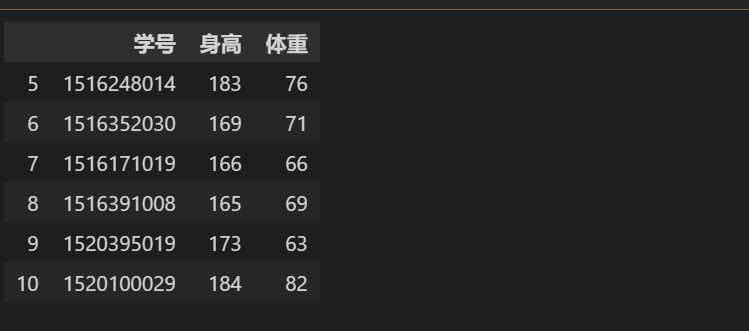```# sql查询语言 身高高于170   性别是女
stu.query('身高 > 170 and 性别 == "女"')
# pandas查询
stu[ (stu['身高'] > 170) & (stu['性别'] ==  "女") ]```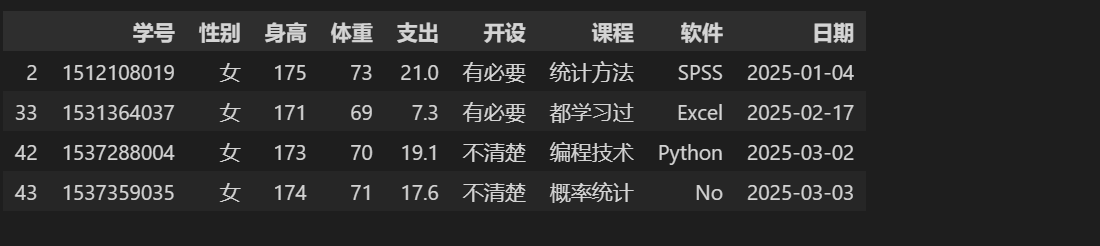```# 通过索引号获取信息
stu.query('10')```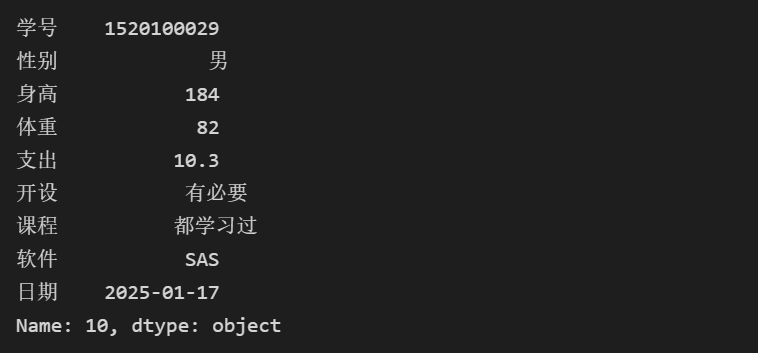```stu['身高'].sort_values() # 默认正序
stu['身高'].sort_values(ascending=False) # 默认正序```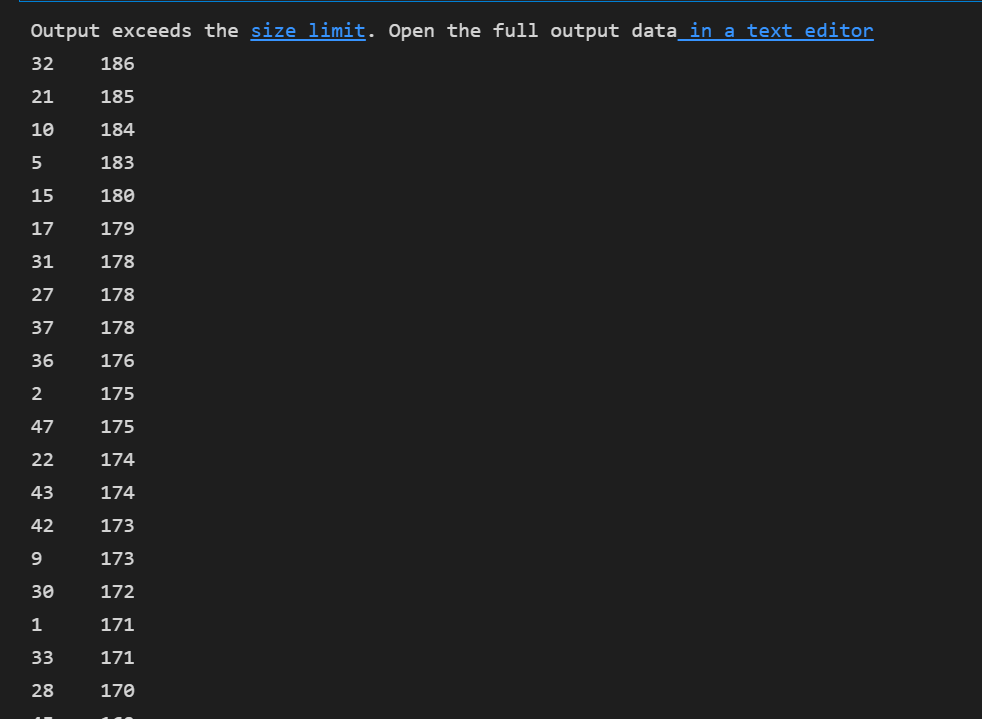```# 按课程分组，查看分组里面的数据
stu = stu.groupby('课程')
stu.groups```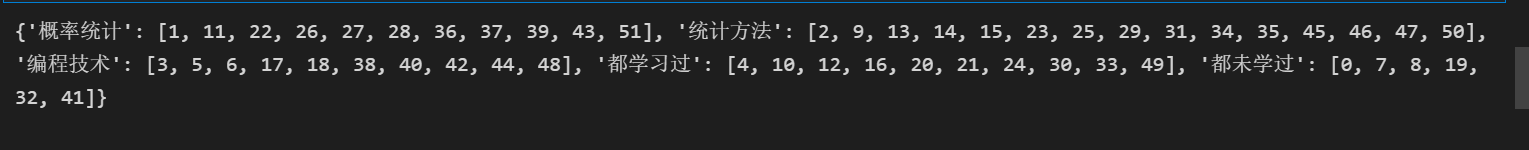```# 查看分组描述
stu.describe()```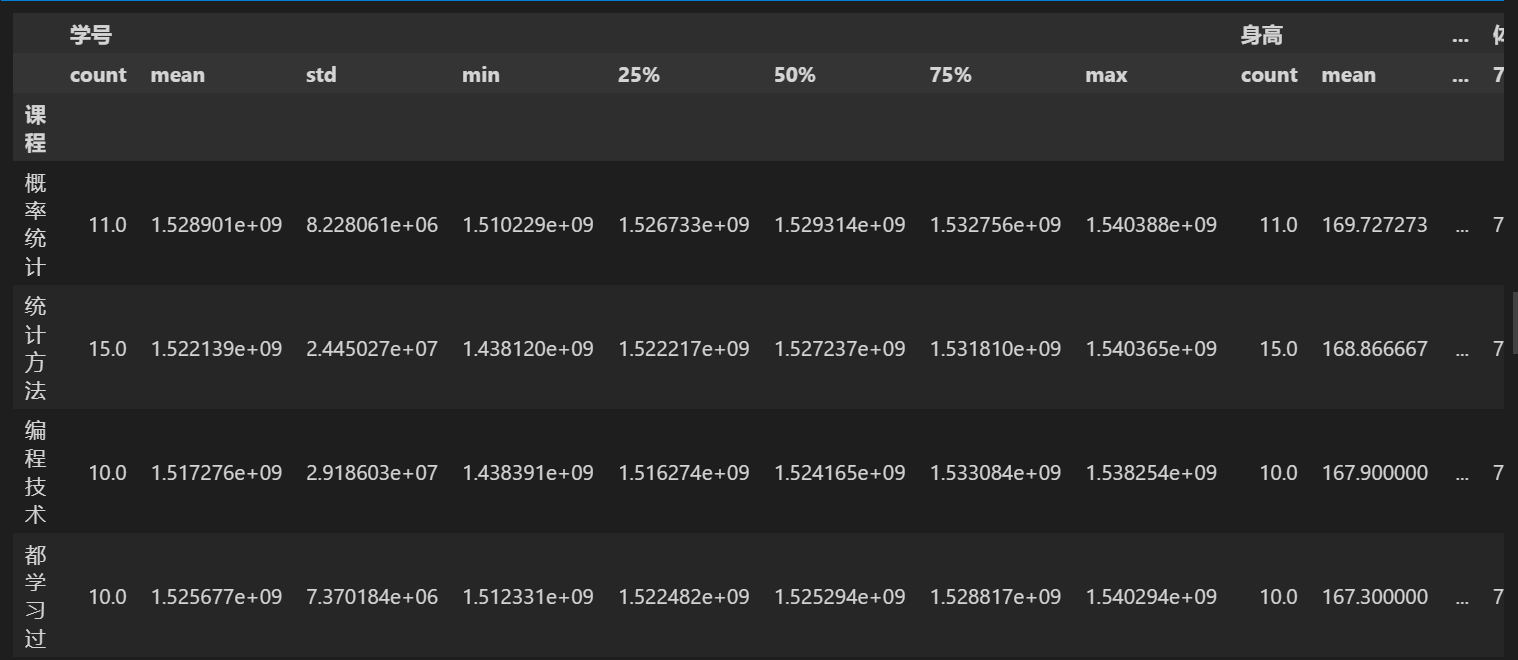```# 分组汇总
# stu.agg(['mean','std']) # 分组后每一列的均值和标准差
print(stu.身高.agg(max))```⭐ 数值变量分段

```stu = pd.read_excel('./stu_data.xlsx')
stu['新体重'] = pd.cut(stu.体重,bins=[40,50,60,70,80,90],right=False)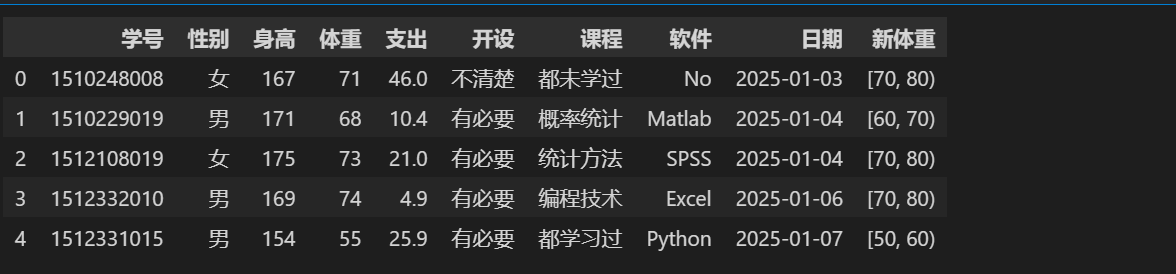```# stu.日期
stu['年份'] = stu.日期.dt.year
stu['月份'] = stu.日期.dt.month
stu['天数'] = stu.日期.dt.day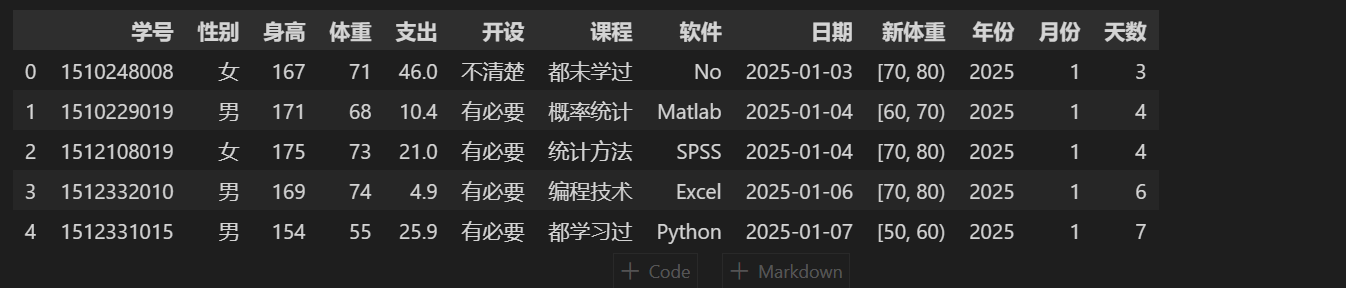```# Period 日期转换想要的格式
stu["日期"].apply(lambda x:pd.Period(x,freq='H')) # 保留到小时
stu["日期"].apply(lambda x:pd.Period(x,freq='M')) # 保留到月份``````# 创建新Series对象
stu1 = pd.Series(np.arange(12345678900,12345678952),name='手机号')
stu1```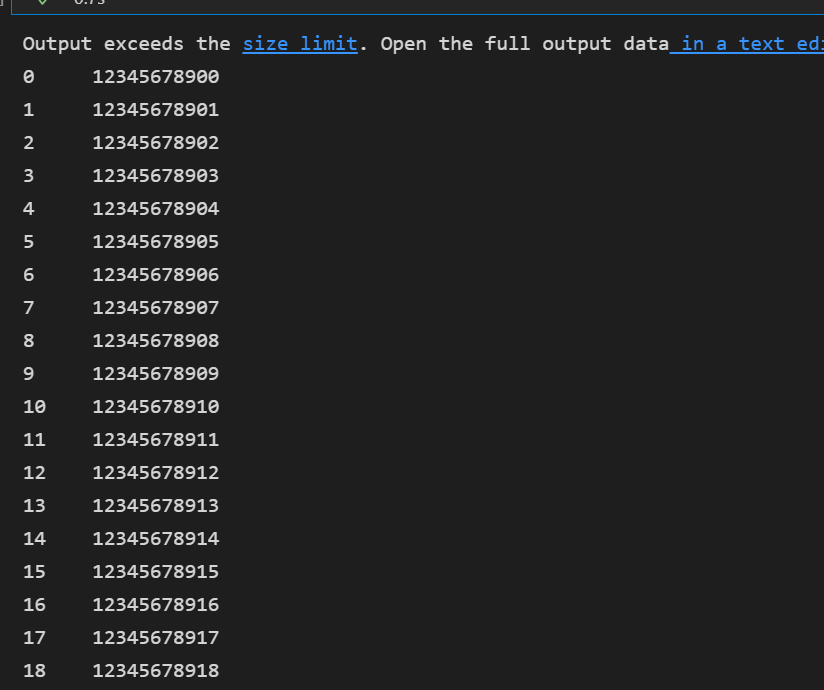```# 合并表stu3 = pd.concat([stu,stu1],axis=1)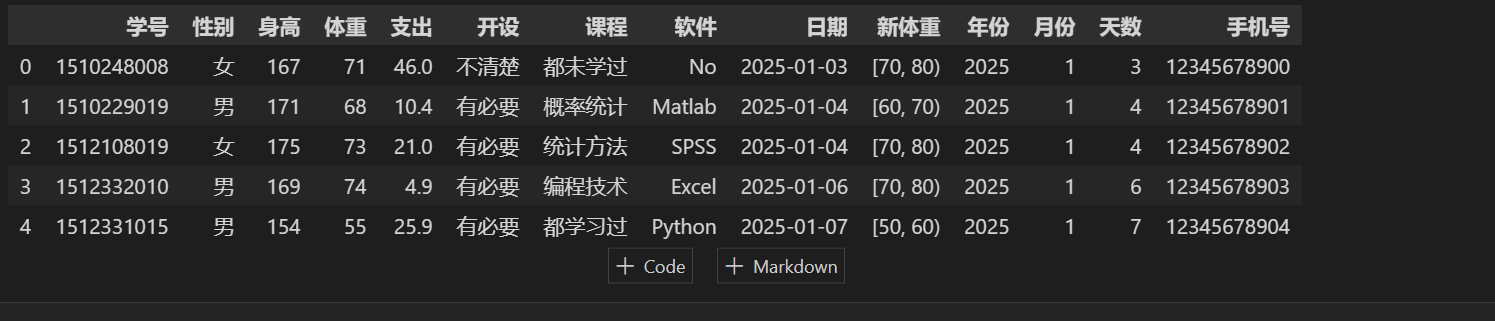` `
```# join表连接
dict1 = {
'employee_id':['NC001','NC002','NC003','NC004'],
'name':['zs','lian','ls','wu'],
'sex':['m','f','f','m'],
'department':['php','python','c++','java'],
'item_id':['N100','N103','N103','N100'],
}
dict2 = {
'item_id':['N100','N102','N103','N104'],
'item_name':['php','python','c++','java'],
'location':['SZ','SH','BJ','GZ']
}
items = pd.DataFrame(dict2)
signup = pd.DataFrame(dict1)
print(signup)
print(items)```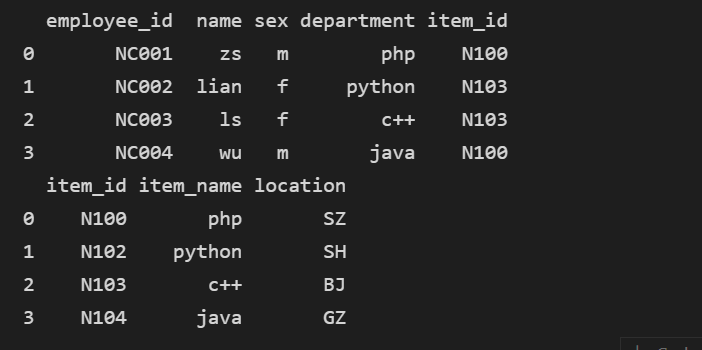```# join合并表，只输出报名人数不为0的项目及其对应的报名人数
df = items.set_index('item_id').join(signup.set_index('item_id'), on='item_id', how='inner')
df.groupby('item_name')['employee_id'].count()````⭐ mode()函数的使用`
```# Pandas dataframe.mode()函数获取沿所选轴的每个元素的模式。
# 获取身高的众数，并返回对应的身高值
import pandas as pd
Nowcoder.loc[:,['身高']].mode()```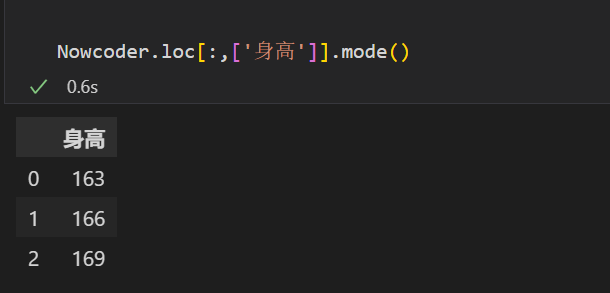⭐ 修改索引

```# 修改索引
```df1.index = ['0','1','2','3']